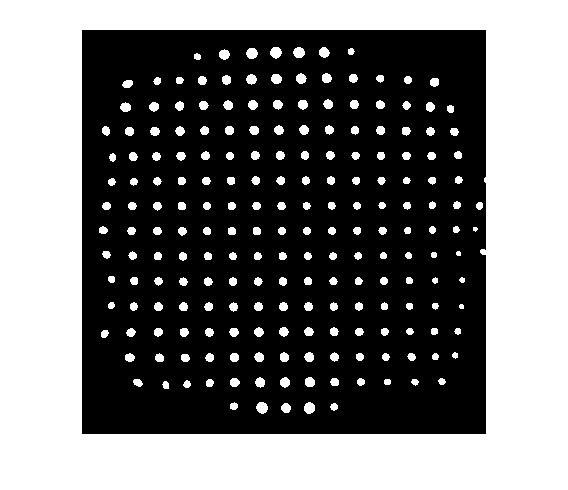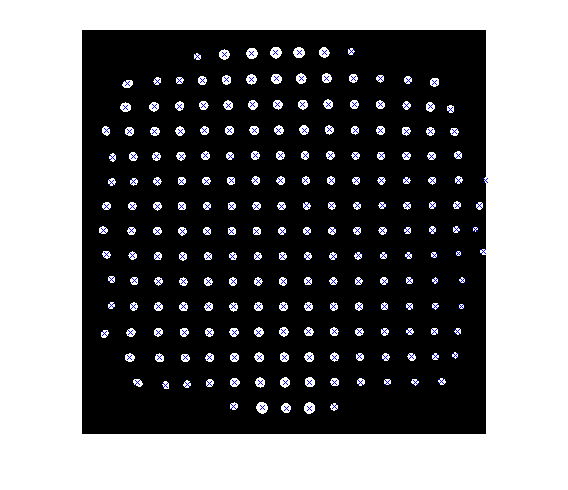# Determining point positions in MRI peg phantom

Blog reader Jonathan from St. Jude Children's Research Hospital sent me an image derived from an MRI peg phantom:

bw = imread('https://blogs.mathworks.com/images/steve/62/mri_peg_phantom.png');
imshow(bw)Jonathan wanted to know how to determine the position of each point. The functions bwlabel andregionprops do the trick.

The function bwlabel takes a binary image and figures which groups of white pixels are connected to each other.

L = bwlabel(bw);

The output L is called a label matrix. It has the same size as bw and contains nonnegative integers. Each positive integer value corresponds to a particular object. For example, to display the 10th object, just compare L to 10:

imshow(L == 10)The function regionprops computes a number of different geometric properties of all the different regions contained in a label matrix. All we need for this application is the centroid:

s = regionprops(L, 'Centroid')
s =

205x1 struct array with fields:
Centroid



The size of the structure array s tells you the number of labeled objects: 205. The centroid of the 10th object is:

centroid_10 = s(10).Centroid
centroid_10 =

44.1687   77.8072



Here's a simple way to superimpose the centroid locations onto the original image:

imshow(bw)
hold on
for k = 1:numel(s)
plot(s(k).Centroid(1), s(k).Centroid(2), 'x')
end
hold offThanks for letting me show this image in the blog, Jonathan.

Published with MATLAB® 7.2

|

### コメント

コメントを残すには、ここ をクリックして MathWorks アカウントにサインインするか新しい MathWorks アカウントを作成します。